# Kinetic Modelling and Optimizing of Butyl Propionate over a Synthesied Material (Tungstan Phosphoric Acid) Heteropoly Catalyst Using Response Surface Technique

Kinetic Modelling and Optimizing of Butyl Propionate over a Synthesied Material (Tungstan Phosphoric Acid) Heteropoly Catalyst Using Response Surface Technique
National Institute of Technology Warangal (NITW), Telangana 506004, India

Corresponding Author Email:
raju.kalakuntala@gmail.com
Page:
273-280
|
DOI:
https://doi.org/10.18280/acsm.450402
21 July 2021
|
Accepted:
19 August 2021
|
Published:
31 August 2021
| Citation

OPEN ACCESS

Abstract:

The performance of heteropoly acid i.e., Tungstan phosphoric acid for the synthesis of butyl propionate at optimized conditions. Effect on conversion and yield of propionic acids using the Response Surface Methodology (RSM) were evaluated by different process parameters including catalyst loading, alcohol/acid molar ratio. There were no external and internal mass transmission limits. A quadratic model acquired by the variance study (ANOVA) has been shown to view experimental data successfully with the regression (R2 = 0.94 and R2 = 0.942) coefficients approaching to unity. The pseudo homogeneous kinetic model (PH) validated with experimental data to determine kinetic parameters i.e., activation energy (45.97 kJ/mol) and frequent factor (91319 L/mol-min).

Keywords:

butyl propionate, optimization, kinetic model, Tungstan phosphoric acid

1. Introduction

Esters have major role in chemical applications, which includes fragrances, additives, surface agents, enamels and flavors. Different forms of esters with different approaches i.e., esterification, Transesterification etherification etc. are developed for various applications. Among this the basic and popular method of producing carboxylic acid and alcohols in presence of catalysts is esterification [1-6].

The Esterification reactions are limiting by chemical equilibrium. The homogeneous catalysts like, H2SO4, HCl and p-toluene Sulfonic acid may have strong catalytic activity, but they have disadvantages of corrosion, side reactions and product purification . To overcome this drawback, it is proposed use heterogeneous catalysts which have multiple benefits i.e., easy separation, high purity and eliminating the corrosion and side reactions, thus heterogeneous catalysts are preferred for Esterification reaction. This includes solid acid catalysts, base, ion exchange resins and supported catalysts etc.

Heteropoly acid catalysts are selective in sorption of reactants and possesses swell up in nature. Tungsten phosphoric acid have high catalytic activity and widely used for Esterification [7-10].

Response surface methodology is an effective mathematical and statistical approach for the experimental design, development of models, optimization of experiments and the development of optimal operating conditions for the system response . It is a time-saving method that allows users to perform a small number of experiments to collect a large amount of data. It also allows the variable effect and combination of interactions on response variable to be studied.

The current research deals with Propionic acid Esterification with n-butanol as a catalyst in the presence of silica supported tungsten phosphoric acid. Multi-response based RSM analysis was used for testing analyzing, and optimizing the effect of different parameters on two variables. Box-Behnken experimental design (BBD) was used to design three essential process parameters, namely catalyst activation, reaction temperature, the molar ratio of acid/alcohol, and two reaction variables, namely Propionic acid conversion and ester yield, and subsequently developed an analytical, computational model relating process variables.

In this analysis, the liquid concentration activities of each component were used to build kinetic model.

Rate determination phase was assumed to be reactive and material sorption on catalyst surface reaction. The second order pseudo-homogeneous (PH) model was assumed and selected under these conditions in order to simulate experimental data.

2. Experimental

2.1 Chemicals

Propionic acid (pure >99%) and n-butanol (pure >99%) were obtained for sigma-Aldrich. Tungsten phosphoric acid catalyst was prepared in laboratory. The catalyst was dried out to eliminate moisture content.

2.2 Preparation of catalyst

The support ZrOwas prepared by precipitation process with aqueous zirconyl nitrate and ammonia solutions. The aqueous nitrate solution is precipitated with drops of ammonia solution by maintaining the pH at 9. Then after complete precipitation, the solution is filtered by using filter paper. The solid mass obtain after filtration was dried at 120℃ in an air oven and subjected to calcinations at 800°C for 4 h. TPA/CeO2 catalysts were prepared by impregnation method . The required amount of TPA is calculated by varying in the range of 5-20%. To prepare these catalysts, appropriate amounts of TPA was dissolved in distilled water. This solution has been supplemented with known quantities of ZrO2. The mixture has been under agitation for 6 hours. Excess water was evaporated and the obtained solid was calcinated at a temperature ramp of 3°C/min for 4 hours.

2.3 Experimental procedure

To evaluate the activity of prepared Tungsten phosphoric acid a standard sample of 1 g of catalyst poured in a conical flask containing 50 cm3 of 1 N solution of sodium chloride and kept in ultrasonic bath for one hour. The obtained solution was titrated against a solution of 0.1 N sodium hydroxide. The ion exchange activity measured by an average of three readings i.e., 5.3 ± 0.05 meq/g, which is consistent with the quality of the supplier.

Three neck flasks with condenser and magnetic agitator were used to perform the batch experiments. The flask was heated with the help of a PID temperature controller. The N-butanol and catalyst are loaded in an RB flask and heated to the required temperature. It is known to be zero when Propionic acid was applied to the flask. Every 15 minutes, samples are removed.

2.4 Analysis

All the experimental runs conducted three times to confirm the reproducibility of findings. The sample of response mix was calculated using phenolphthalein as an indicator for titrating with a solution of 0.1 N sodium hydroxide. (Karl Fischer titrates based on a microprocessor (Optics Technology, India) evaluated the reaction mixture's. It was verified that no side reactions were occurred after ensuring the water content was measured.

2.5 Characterization of the catalyst

2.5.1 UV-visible

Figure 1 shows the Diffuses reflectance’s of UV-visible spectra of tungsten phosphoric acid. From the figure the adsorption bands at 254 and 315 nm are attributed to the tungsten charge adsorption bands for the kegging anion changes in intensities of the of these bands due to the presence of other component like silica supports ).

## 1.pngFigure 1. UV visible spectra of tungsten phosphoric acid

2.5.2 FTIR

Figure 2 shows the FTIR spectra of the tungsten phosphoric acid. Some of the important bands are observed in the spectra are 801cm-1 (symmetric stretching frequency of Si-O-Si), 889cm-1 (symmetric stretching frequency of H-O), 893cm-1 (symmetric stretching frequency of H-O-H) and 1081cm-1 (symmetric stretching frequency of Si-O-H). peaks in the region of 3500cm-1 are observed which corresponds to the adsorbed water in the system. Peak bands observed between 889 and 1081 conforms it is tungsten phosphoric acid .

## 2.jpg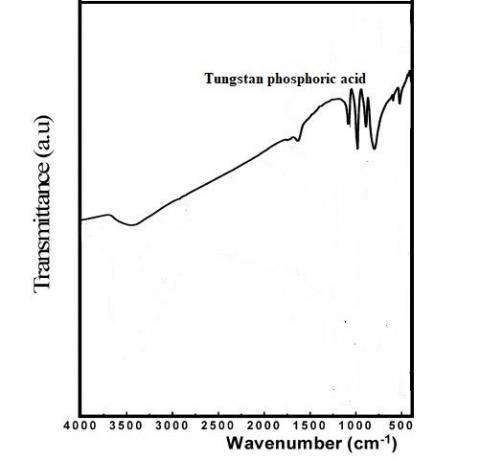Figure 2. FTIR spectra of tungsten phosphoric acid

2.6 Results and discussion

2.6.1 Mass transfer considerations

Mass transfer limitations in the proposed esterification reaction was evaluated by conducting experiments at different e stirrer speeds of 240, 480 and 720 rpm at working temperature of 388°K temperature with catalyst load of 1%. Figure 1 shows that there was no significant difference in response rate followed by a propionic acid conversion by adjusting the stirrer speed. The differences were considered negligible, particularly the stirrer speeds over 480 rpm. This indicated the absence of internal mass transfer resistance over 480 rpm and hence all experiments were carried out at a stirrer speed of 480 rpm.

Table 1. Three level variables for coded values

 Variables Symbols Coded Values -1 0 1 Catalyst Loading X1 1 2 3 Mole Ratio (Acid to Alcohol) X2 1 2 3 Reaction temperature(°K) X3 368 378 388

2.6.2 Experimental design and mathematical model

Response Surface (RSM) methodology was used for the analysis of reaction parameters for esterification to achieve a major change in propionic acid for higher rate of ester production. Table 1 shows three-level system variables, including catalyst charging (X1), acid to alcohol mole ratio (X2), and reaction temperature(X3) to establish an experimental design. The propionic acid (Y1) and ester production (Y2) percentage conversion were selected as the Experimental design response variables. The standard Experimental design of Box-Behnken applied to conduct Seventeen sample sets (12 factorial and 5 middle points). Design Expert Version 10.0.6 was used to model and test the experimental data.

A polynomial second order expression suggested by RSM was used to evaluate the best value to expose the relations between the variables. The equation has been expressed as:

$Y=\beta_{0}+\sum_{i=1}^{k} \beta_{i} X_{i}+\sum_{i-1}^{k} \beta_{i i} X_{i}^{2}+\sum_{i=1}^{k} \beta_{i j} X_{i} X_{i j}+e$

where, Y denotes response variable, Xi indicates independent coded concentration variables. βo, βii, βii, βij reflects the coefficients of regression, linear terms, square interaction conditions between i and j, respectively. Xi, Xii, and Xij denote Linear conditions, quadratic and interactive coded variables conditions. K is the number of variables used to maximize the propionic acid conversion and e is a suspected error.

2.7 RSM analysis

2.7.1 Model determination

Table 2. Statistical analysis by the design expert

 Model Sum of Squares R2 Adjusted.R2 Suggestions Linear 0.0063 0.4505 0.3237 Not suggested 2FI 0.0059 0.4852 0.1763 Not suggested Quadratic 0.0007 0.94 0.863 Suggested Cubic 0.0000 -- -- Aliased

The statistical analysis is provided by the Design Expert program in Table 2. The values R2 and adj-R2 for linear relations are respectively 0.4505 and 0.323, which indicates that linear relations are not appropriate for experimental information. Despite the reduction in the R2 and Adj-R2, the code suggests a quadratic model, as the cubic model is aliased. The quadratic model has therefore been selected to match the experimental results.

2.7.2 Model adaptation and variance analysis (ANOVA)

Experiments are randomly carried out to prevent distortion with the center-united Box-Behnken format (BBD). Table 3 displays findings after 17 fixed reaction time studies of 4 hours. The best fit is determined by a multi-regression and backward removal. The conversion and yield values for propionic acid are also shown in Table 3.

The quadratic relation between system and variables of the second order is defined as based on RSM evaluation:

$Y_{1}=42.18+0.077 X_{1}-0.072 X_{2}+0.233 X_{3}-0.012 X_{1}^{2}+0.008 X_{2}^{2}-0.0003 X_{3}^{2}-0.0097 X_{1} X_{2}$

$+0.0002 X_{2} X_{3}+0.000025 X_{1} X_{3}$

$Y_{2}=0.9094+0.0195 X_{1}+0.0168 X_{2}+0.004 X_{3}-0.0123 X_{1}^{2}+0.009 X_{2}^{2}-0.0326 X_{3}^{2}$

$-0.01 X_{1} X_{2}+0.0021 X_{2} X_{3}+0.0003 X_{1} X_{3}$

Table 3. Propionic acid conversions and yield of esters with obtained model
 Run Experimental Variables Conversion of propionic acid The yield of ester (%) X1 X2 X3 Experimental Predicted Experimental Predicted 1 3 3 368 0.929 0.933 0.927 0.932 2 2 1 358 0.872 0.869 0.869 0.867 3 2 2 368 0.911 0.911 0.909 0.909 4 2 2 368 0.911 0.911 0.909 0.909 5 3 2 358 0.892 0.882 0.889 0.879 6 2 3 378 0.908 0.91 0.906 0.908 7 1 2 358 0.838 0.844 0.834 0.841 8 1 3 368 0.927 0.914 0.925 0.913 9 2 2 368 0.911 0.911 0.909 0.909 10 2 1 378 0.879 0.873 0.876 0.870 11 2 3 358 0.893 0.898 0.876 0.896 12 2 2 368 0.911 0.911 0.909 0.909 13 3 2 378 0.897 0.890 0.895 0.888 14 2 2 368 0.911 0.911 0.909 0.909 15 1 2 368 0.842 0.851 0.838 0.848 16 1 1 368 0.867 0.862 0.864 0.859 17 3 1 368 0.908 0.92 0.906 0.918

Table 4. ANOVA results for obtained for the conversion of propionic acid

 Sources Sum of squares Degrees of freedom Mean square F-value P-value Characterization Model 0.0113 9 0.0113 12.2 0.0017 Significant X1 0.003 1 0.003 29.48 0.001 X2 0.0023 1 0.0023 21.90 0.0023 X3 0.0001 1 0.0001 1.23 0.3047 X12 0.0006 1 0.0006 6.19 0.0417 X22 0.0003 1 0.0003 3.29 0.112 X32 0.0045 1 0.0045 43.33 0.0003 X1X2 0.0004 1 0.0004 3.88 0.0895 X2X3 0.0000 1 0.0000 0.1633 0.6982 X1X3 2.63E-07 1 2.63E-07 0.0026 0.9611 Residual 0.0007 7 0.0001 Lack of fit 0.0007 3 0.0002 Pure error 0.0000 4 0.0000 Cor Total 0.012 16 R2=0.94; Adjusted R2=0.863 Predicted R2=0.855; Adequate precision=11.69

Table 5. ANOVA results for obtained model for the yield of ester

 Sources Sum of squares Degrees of freedom Mean square F-value P-value Characterization Model 0.011 9 0.0119 12.4 0.0018 Significant X1 0.003 1 0.003 29.48 0.001 X2 0.002 1 0.0023 21.90 0.0023 X3 0.0001 1 0.0001 1.23 0.3047 X12 0.0006 1 0.0006 6.19 0.0895 X22 0.0003 1 0.0003 3.29 0.9611 X32 0.0045 1 0.0045 43.33 0.6982 X1X2 0.0004 1 0.0004 3.88 0.0417 X2X3 2.63*10-7 1 2.63*10-7 0.1633 0.1126 X1X3 0 1 0 0.0026 0.0003 Residual 0.0007 7 Lack of fit 0.0007 3 Pure error 0 4 Cor Total 0.012 16 R2=0.94; Adjusted R2=0.863 Predicted R2; Adequate precision=11.69

Propionic acid transformation and yield increases linearly due to the positive signal coefficients i.e., synergistic effects, whereas negative sign coefficients show antagonistic effects. It has been noted from Equations (2 and 3) that the catalyst loading linear coefficient (X1) has the most significant value of the linear coefficient, among other variables. This showed the major consequences of catalyst charging for the conversion and yield of propionic acid. The effect on propionic acid conversion and ester yield is the ratio of alcohol with molar acid (X2) followed by a lower value (X3). Parameter interaction (X1X2), though parameter (X1X3) and parameter (X2X3) have observed much weaker interaction effects. This is due to the combined impacts of response parameters, comparable findings published in the literature .

The ANOVA results from the model obtained are shown in Tables 4 and 5. Compared the mean square with the forecast fault, ANOVA gives the statistical value of each effect for a number of experiments. The reliability of the model was also measured by the variance coefficient and the F-value test. The mean square relation between the model and the medium square error is shown in Tables 4 and 5 of F values.

The study of the F-value uses p-value to assess the significance of regression coefficients. 12.02 or 12.20 represented a 0.01% risk of high F value due to noise for that model. The p-value <0.05 shows the conditions of the model. In this case, the words of the model are fundamental to all linear, quadratic and interaction results. The determination ratio (R2) of 0.94 and 0.942 showed that 94 and 94.2 per cent of variance could be described by models. The R2 values 0.855 and 0.863 foreseen are consistent with the 0.863 and 0.869 for changed R2 values. The Signal to Noise Ratio indicates the Adequate Precision value. In particular, the rate higher than 4 for optimal design effectively. The proportions of 32.361 and 33.735 give an acceptable Signal in the current case, therefore it can be used to access the model rooms .

2.7.3 Model accuracy check

For an appropriate model, the precision test is important. The predicted experimental values were checked by comparing them with the model values. In Figure 3 the predicted and experimental values are strongly linearly correlated between propionic acid conversion and the ester production.

A similar residual plot was nevertheless obtained. Internally analyzed residues determine the standard deviations from the estimated experimental values. A stable linear link between normal probability (percentage) and the residuals studied internally indicated the need for a reaction change and no strong normalcy issue.

## 3.jpgFigure 3. Actual vs predicted values of (a) propionic acid conversion

2.8 Response analysis

Figures 4-9 indicate that three parameters are chosen to be linked between answer variables. Each plot displays interactions of two reaction variable parameters within their scope, retaining another zero-point variable. The response surfaces of model better display trend towards conversion of each factor. The 3D reaction surface and 2D contour plots were developed with equations (2 and 3). The contour plot type demonstrates the essence and extent of relationships with parameter. The interaction between the respective parameters becomes prominent if the contour plot is circular, when it became negligible.

The effect of Relationship among Catalyst Loading and molar acid/alcohol ratio on propionic acid converting, an increase in propionic acid conversion could lead to an increase in catalyst quantities. Propionic acid conversion has also improved with the increased molar ratio. The loading of catalysts and molar ratio has major effect on change of propionic acid. Lower P-value of this result (<0.0001) is supported by X1X2 (Table 4) interface term, and positive X1 and X2 Linear terms coefficients. Intricate molar ratio of propionic acid vs n-butanol is calculated by taking into account reaction temperature and catalyst concentration. In equilibrium, the molar ratio increases with the addition of n-butanol, which increases the propionic acid conversion rate.

Figure 5 shows impact of these parameters on ester output. The contact between catalyst loading, molar ratio was noted as powerful effect on ester output. The elevated loading of the catalyst with more reactants improves the rate of mass transfer, which consequently enhances the Output of the product. The contour plot elliptical shape and very small p-value (<0.0001) of contact word X1X2 (Table 5) reveal the meaning of this interaction effect. The experiments conducted at different temperatures indicate that the rate of propionic acid conversion increases with temperature. The reaction reaches equilibrium at a given temperature. However, at lower temperature, it takes longer to reach equilibrium than at higher temperature.

## 4.png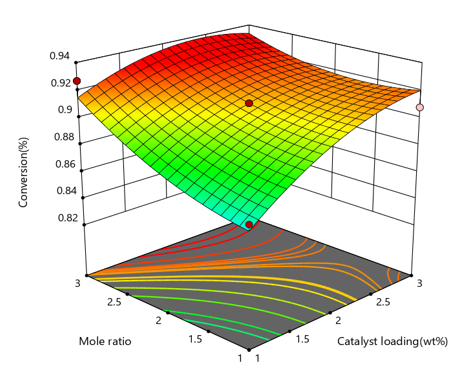Figure 4. Response surface plot for the effect of molar ratio and catalyst loading on conversion of propionic acid with temperature at the zero level

## 5.png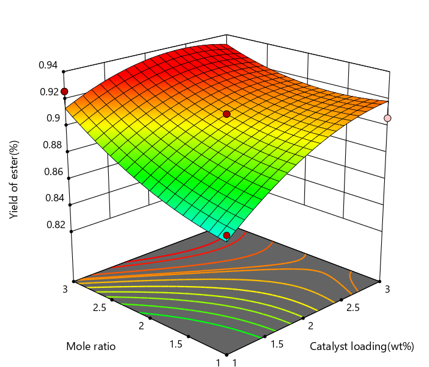Figure 5. Response surface plot for the effect of molar ratio and catalyst loading on yield of ester with temperature at the zero level

## 6.png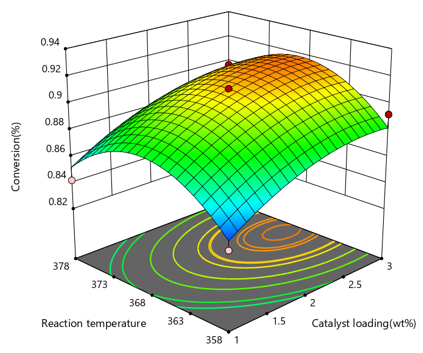Figure 6. Response surface plot for the effect of reaction temperature and catalyst loading on conversion of propionic acid with mole ratio at the zero level

Figure 6 explains the impacts on propionic acid conversion by different catalyst load and reaction temperature. The temperature of sample plays an essential part in determining esterification reaction rate. From Figure.6, it is evident that at reduced temperature, catalyst enhancements bring higher propionic acid conversion. However, there was no important rise in propionic acid conversion at a higher temperature. This could be due to the slightly greater temperature deactivation of the catalyst.

The contact between catalyst loading with temperature on ester results shown in Figure 7. Improving catalyst quantity and temperature increases ester yield, but at higher temperatures, a marginal increase in yield was observed. A rounded contour plot and reduced P-value (0.0017) of contact term X1X3 (Table 5) show’s effect of catalyst loading and temperature.

## 7.png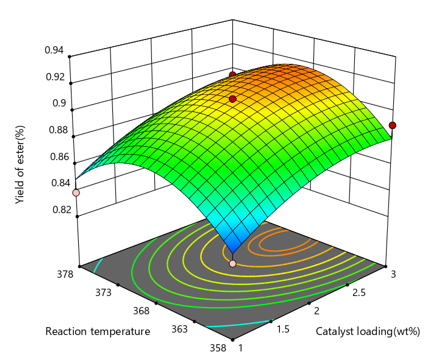Figure 7. Response surface plot for the Effect of reaction temperature and catalyst loading on yield of ester with mole ratio at the zero level

Figure 8 shows impacts on propionic acid conversion with different molar ratio’s of acid/alcohol and reactant temperature. Figure shows change of Propionic acid improved uniformly, at any temperature from 358oK to 398oK with molar ratio. This implies that at distinct molar ratios, the temperature of the response displayed less impact on the conversion. These results validated the reduced value of the Linear term equation coefficient. The contour plot demonstrates these two variables prominent interactions .

## 8.png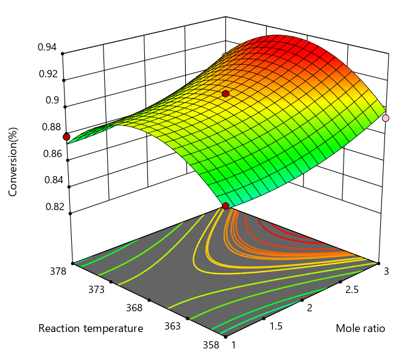Figure 8. Response surface plot for the Effect of reaction temperature and mole ratio on conversion of propionic acid with catalyst loading at the zero level

Figure 9 shows the relationship between the molar acid-alcohol proportion and the ester output temperature. The rise in the molar ratio improves the output of the product in proportion to the temperature rise. However, very slight increases in ester output were observed at a higher temperature. The Effects of two parameters was found significant with elliptical nature of contour curve and reduced p-value (0.0010) of X2X3.

## 9.png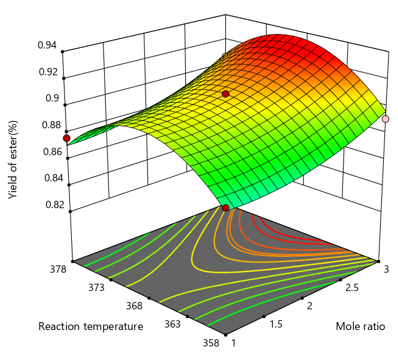Figure 9. Response surface plot for the Effect of reaction temperature and mole ratio on yield of ester with catalyst loading at the zero level

Optimization of Process Parameters

optimal values for chosen parameters have been evaluate by mathematical model by Design Expert software version 10.0.6. Catalyst loading, acid/alcohol molar ratio and the temperature in reaction coded from low (-1) to low (+1) are the parameters selected in optimization (Table 3), whereas propionic acid and ester convert to optimize minimal to maximum outputs (see Table 3). The best approach to check the model prediction was chosen. The optimum conditions for propionic acid conversion and ester yield, including predicted and experimental values. The experimental value given in Table 6 is an average of empirical data that are consistent with expected values. Propionic acid and N-butanol esterification was optimized with a statistically adequate methodology of the Box-Behnken configuration and the quadratic model.

Table 6. An average of empirical data that are consistent with expected values

 Parameters X1 X2 X3 Y1 (conversion) Y2 (Yield) Predicted 2.35 2.9 365.5 0.931 0.927 Experimental 2 2 368 0.911 0.909

2.9 Kinetic model

The propionic acid (PA) esterification response with N-butanol (NB) for the production of butyl propionate (BP) and water (W) in the presence of Tungsten phosphoric acid catalyst.

$\mathrm{PA}+ \mathrm{NB} \leftarrow \quad --- \quad \rightarrow \mathrm{BE}+\mathrm{W}$

Under optimized operating conditions (Table 6) independent experiment was conducted for kinetic study and results shown in Figure 10. There was a lack of internal and external constraints on mass transfer. Butyl propionate (BP) formation may be split into three separate steps.

$\mathrm{PA}+\mathrm{C} \leftarrow \quad\quad------\quad\quad \rightarrow$ PA.C;

$-r_{1}=k_{1} C_{P A} C_{C}-k_{-1} C_{P A} C_{C}$

$\mathrm{PA} . \mathrm{C}+\mathrm{NB} \leftarrow\quad\quad------\quad\quad \rightarrow \mathrm{BE.C}+\mathrm{W}$

$-r_{2}=k_{2} C_{P A . C} C_{N B}-k_{-2} C_{B E . C} C_{W}$

BE.C $\leftarrow \quad\quad-----\quad\quad\rightarrow \mathrm{BE}+\mathrm{W}$

$-r_{3}=k_{2} C_{B E . C} C_{N B}-k_{-3} C_{B E} C_{C}$

$-r_{a}=-r_{2}=\frac{k_{2} K_{1} C_{t}\left(C_{P A . C} C_{N B}-\frac{k_{-2} C_{B E . C} C_{W}}{K_{e q}}\right)}{\left(1+k_{1} C_{P A}+k_{-3} C_{B E}\right)}$

$K_{e q}=\frac{C_{B E} C_{W}}{C_{P A} C_{N B}}=\frac{X_{e q}^{2}}{\left(1-X_{e q}\right)\left(M-X_{e q}\right)}$

$-r_{a}=-r_{2}=k_{a}\left(C_{P A . C} C_{N B}-\frac{k_{-2} C_{B E . c} C_{W}}{K_{e q}}\right)$

$k_{a}=k_{2} K_{1} C_{t}$

The steady reaction rate, K1 can be calculated using Equation for the information acquired at optimized process circumstances using the regression technique. Equation was also used to calculate the reaction rate using derived equation. Figure 10 shows a parity plot calculated by experiment and predicted data.

Figure 11 shows the experimental statistics were excellent agreement with data predicted by the model. The active line is a fit curve (R2 = 0.952), and the dots are confidence interval of 95%. Also, the change in component concentration under optimized process conditions was calculated and plotted against time using the kinetic model equation.

## 10.png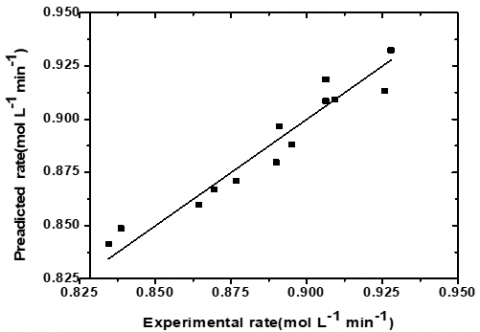Figure 10. Parity plot of experimental vs predicted rate

Figure 11 shows that the findings predicted by the experiment and model are in excellent agreement. This indicates that for the esterification reaction, the PH kinetic model is valid.

Figure 12: P-H kinetics model validation the equation Arrhenius is used to expressing the response temperature dependence and expressed as equation. The rate constants were achieved using the kinetic model under optimized working circumstances at distinct reaction temperatures (including optimized response temperature).

## 11.png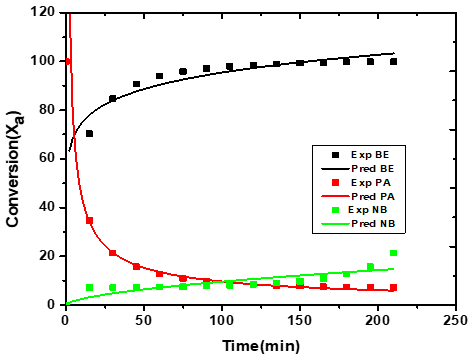Figure 11. Conversion vs time with experimental and simulated data

## 12.pngFigure 12. Arrhenius plot

A plot of ln(ka0) vs .1/T is shown in Figure 12 indicate a straight line. The energy of activation (E) and pre-exponential actor (ki) were evaluated using software Origin Pro 8.

$\ln k=\ln k_{i}^{0}-\frac{E_{i}}{R T}$

The energy of activation and pre-exponential factors for esterification reaction are 45.97 kJ/ mol and 91319 L/ mol-mi. Diffusion-limited responses have been recorded to demonstrate low power activation. Thus, the high activation energy (45.97 kJ/mol) shows that propionic acid and N-butanol esterification is kinetically controlled in the current research.

3. Conclusions

At the stirrer velocity of as much as 480 rpm, internal mass transfer resistance was absent. The inner restriction on the transfer of mass was experimentally evaluated and discovered to be missing. A quadratic model was significant for esterification response and fit experimental data with a regression coefficient (R2 = 0.94 and R2 = 0.942) and an Adj-R2 of (0.863 and 0.869) values. In addition, this model's p-value was < 0.0001, which disclosed a significant model. At optimum process circumstances (2.35 catalyst load (percent wt/wt), 2.9 molar ratio (acid to alcohol), and 365.5oK response temperature, 93.1 percent, and 92.7 percent were anticipated for conversion and yield. Experiments were conducted to verify model's validity, accuracy and outcome shows predicted value was similar to experimental values (91.11 ± 0.021% and 90.909±0.019). In addition, kinetics was studied, and preliminary data were simulated using the second-order pseudo homogeneous (PH) model. The experimental findings were well anticipated by the kinetic model established. Due to high activation energy, 45.97 kJ/mol esterification response discovers kinetically controlled, and the frequency factor is 91319 L/mol-min.

References

 Chandane, V.S., Rathod, A.P., Wasewar, K.L., Sonawane, S.S. (2017). Process optimization and kinetic modeling for esterification of propionic acid with benzyl alcohol on ion-exchange resin catalyst. Korean Journal of Chemical Engineering, 34(4): 987-996. https://doi.org/10.1007/s11814-017-0006-4

 Sofia, L.A., Krishnan, A., Sankar, M., Kala Raj, N.K., Manikandan, P., Rajamohanan, P.R., Ajithkumar, T.G. (2009). Immobilization of phosphotungstic acid (PTA) on imidazole functionalized silica: evidence for the nature of PTA binding by solid state NMR and reaction studies. The Journal of Physical Chemistry C, 113(50): 21114-21122. https://doi.org/10.1021/jp906108e

 Chandane, V.S., Rathod, A.P., Wasewar, K.L., Sonawane, S.S. (2016). Esterification of exchange resins: Optimization and kinetics. Korean Journal of Chemical Engineering, 34: 249-258. https://doi.org/10.1007/s11814-016-0249-5

 Yan, X., Yan, J., Mei, P., Lei, J. (2008). Synthesis, characterization and catalytic properties of mesoporous HPMo/SiO2 composite. Journal of Wuhan University of Technology-Mater. Sci. Ed., 23(6): 834-838. https://doi.org/10.1021/ie049157g

 Kalakuntala, S.S.R., Navya, R., Sisira, T. (2018). Experimental studies on reactive distillation of propionic acid using n-butanol as entrained. International Journal of Engineering and Technology (UAE), 3(29): 46-48. https://doi.org/10.14419/ijet.v7i3.29.18459

 Raju, K., Babu, G.U.B., Surnanai, S. (2018). Heat and mass transfer limitations in esterification of propionic acid over ion exchange resin. International Journal of Mechanical and Production Engineering, 6(5): 51-54.

 Lotero, E., Liu, Y., Lopez, D.E., Suwannakarn, K., Bruce, D.A., Goodwin, J.G. (2005). Synthesis of biodiesel via acid catalysis. Industrial & Engineering Chemistry Research, 44(14): 5353-5363. https://doi.org/10.1021/ie049157g

 Shu, Q., Nawaz, Z., Gao, J., Liao, Y., Zhang, Q., Wang, D., Wang, J. (2010). Synthesis of biodiesel from a model waste oil feedstock using a carbon-based solid acid catalyst: Reaction and separation. Bioresource Technology, 101(14): 5374-5384. https://doi.org/10.1016/j.biortech.2010.02.050

 Na, S., Zhang, M., Dong, X., Wang, L. (2019). Preparation of sulfonated ordered mesoporous carbon catalyst and its catalytic performance for esterification of free fatty acids in waste cooking oils. RSC Advances, 9(28): 15941-15948. https://doi.org/10.1039/c9ra02546d

 Ng, E.P., Goh, J.Y., Ling, T.C., Mukti, R.R. (2013). Eco-friendly synthesis for MCM-41 nanoporous materials using the non-reacted reagents in mother liquor. Nanoscale Research Letters, 8(1): 1-8. https://doi.org/10.1186/1556-276X-8-120